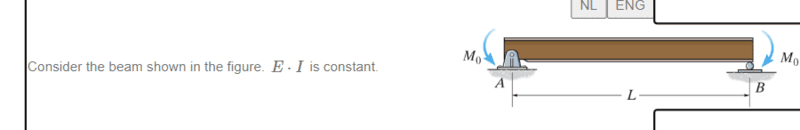# How to solve this problem by the method of superposition?

• Engineering
In summary, the problem is that the table doesn't seem to be very helpful in understanding how to incorporate the moment at the pin into the deformation. However, by reversing the direction of the moment, the response will be reversed.

Homework Statement
Please refer to the picture for a visual.

QUESTION 1: Determine the slope of the beam at A , measured counterclockwise from the positive x -axis.

QUESTION 2:
Determine the maximum deflection of the beam.
(Express your answer in terms of some or all of the variables L, E, I and M_0 in both cases .)
Relevant Equations
Table of deformation --> attachment to be used to determine the maximum deformation + slope via the method of superpositionHello there,

I would like to get some help for this problem if possible.

The problem here is that, after looking at the table I don't really understand how I'd be able to incorporate the moment at the pin A into the deformation. as if you look at the first page 2nd block, there's that simply supported beam, but with a moment that is applied at the side of the roller.

So there are actually two questions here:
(1) for the pin: How do I determine the deformation due to the moment at A
(2) for the roller: the moment is in the opposite direction than shown in the table, does that imply that I could just take the opposite of the equation that is stated next to it for the slope and the deflection?

a note: I do know that the elastic curve will be a 'parabolic' being concave down and deforming above the non-deformed state of the beam, but that is about it on this one.

#### Attachments

• Deform.pdf
534.1 KB · Views: 83
(1) For this case the pin and the roller represent identical support conditions. If your deflection table only supplies results for a moment acting at the right end of the beam, you can use these results for a moment acting at the left end by imagining viewing the beam from the back side.
(2) Reversing the direction of an applied load simply causes a reversal in sign in the response.

•